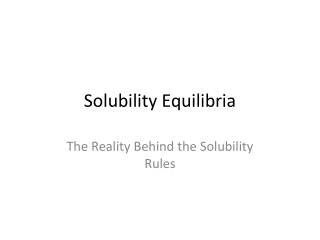DownloadDownload PresentationSolubility Equilibria

# Solubility Equilibria

Download Presentation## Solubility Equilibria

- - - - - - - - - - - - - - - - - - - - - - - - - - - E N D - - - - - - - - - - - - - - - - - - - - - - - - - - -
##### Presentation Transcript

1. Solubility Equilibria The Reality Behind the Solubility Rules

2. Solubility Rules- Qualitativesolubility Equilibrium- Quantitative • Stating that the insoluble salts from the solubility rules do not dissolve is not entirely accurate. Very minute quantities of these “insoluble” salts do in fact dissolve, and the amount that dissolve does so completely, as they are ionic compounds and therefore strong electrolytes.

3. AgCl (s)  Ag+ + Cl-1 • Keq = [Ag+][Cl-1] = Ksp • This is known as the solubility product constant. Same as other equilibrium expressions except that the reactants are solid and do not appear. Product of constituent ions raised to their respective powers.

4. MgF2 Mg+2 + 2 F-1 • Ksp = [Mg+2][F-1]2 • Ca3(PO4)2 • Ksp = [Ca+2]3 [PO4-3]2

5. Ksp from molar solubility • The solubility of Ag2SO4 is 1.5 x 10-2 M, find the Ksp for silver sulfate. Ag2SO4 2 Ag+ + SO4-2 Ksp= [Ag+]2 [SO4-2] Assume 1.0 L of solution, use stoichiometry to determine concentration of each ion, solve for Ksp.

6. 1 mole of Ag2SO4 yields 2 Ag+ ions and 1 SO4-2 ion. • 1.5 x 10-2 M Ag2SO4 x 2 Ag+= 3.0 x 10-2M 1 Ag2SO4 1.5 x 10-2 M Ag2SO4 x 1 SO4-2= 1.5 x 10-2M 1 Ag2SO4 Ksp= [Ag+]2 [SO4-2] Ksp = (3.0 x 10-2)2(1.5 x 10-2) Ksp= 1.4 x 10-5

7. Solubility can also be given in g/L, so we must convert g/L to mol/l and proceed as before. • The solubility of CaSO4 is 0.67 g/L find Ksp. • 0.67g x 1 mol CaSO4 = 4.9 x 10-3mol/L L 136.2 g CaSO4 Ksp = [Ca+2][SO4-2] = (4.9 x 10-3)2 = 2.4 x 10-5

8. More importantly we can use the equilibrium to find the solubility of a salt, and the concentration of the ions at Eq. • Find the molar solubility of CuI. • CuI(s)  Cu+ + I-1 Ksp = [Cu+][I-1] let s = solubility CuI CuI(s)  Cu+ + I-1 Some 0.0 0.0 -s +s +s 5.1 x 10-12 = [Cu+][I-1] = (s)(s) (5.1 x 10-12)1/2 = s = 2.26 x 10-6 mol/L

9. Find the solubility of PbF2 at 25 C. • Ksp = 7.1 x 10-7 • PbF2 Pb+2 + 2F-1 s = [Pb+2] Some +s +2s • 7.1 x 10-7 = [Pb+2][F-1]2 = (s)(2s)2 7.1 x 10-7 = 4s3 5.62 x 10-3 = s = [Pb+2] 1.12 x 10-2 = 2s = [F-1]

10. Q tests • Ksp is an equilibrium constant and we can use it to determine if the salt will still dissolve or will it precipitate. To do this we use a Q-test and compare to Ksp. • Q < Ksp no ppt, unsaturated solution • Q = Ksp no ppt, saturated solution • Q > Ksp ppt appears, supersaturated • Used primarily when mixing solutions

11. When 200 mL of 0.0040 M BaCl2 is mixed with 600 mL of 0.0080 M K2SO4, will a precipitate form? • Write net-ionic for ppt rxn. • BaSO4 Ba+2 + SO4-2 • Ksp = 1.1 x 10-10 • Find [] of each ion [Ba+2] = (0.0040 M)(0.200 L) = 0.0010 M 0.800 L [SO4-2] = (0.0080 M)( 0.600 L) = 0.006 M 0.800 L

12. Ksp = [Ba+2][SO4-2] • Q = (0.0010)(0.0060) = 6.0 x 10-6 • 6.0 x 10-6 > 1.1 x 10-10 so a precipitate forms.

13. Fractional precipitation • Sometimes we can use the solubility and Ksp to separate mixtures of two or more ions. • To separate a mixture of Ba+2 and K+ ions, remove the Ba+2 by adding SO4-2 ions, and filtering the BaSO4 that precipitates. • With two insoluble products, the process is more tedious. Ppt one ion first, filter, and then ppt the other.

14. A solution is 0.020 M in both Cl-1 and Br-1. • What ion will precipitate both of these? • Find the [Ag+] required to initiate ppt of AgBr and AgCl. • Ksp AgBr = 7.7 x 10-13 • Ksp AgCl = 1.6 x 10-10 • AgBr will ppt first AgBr  Ag+ + Br-1 • 7.7 x 10-13 = [Ag+][Br-1] = [Ag+](0.020) • 3.85 x 10-11 = [Ag+] to initiate ppt AgBr

15. For AgCl AgCl  Ag+ + Cl-1 1.6 x 10-10 = [Ag+] [0.0200] 8.0 x 10-9 = [Ag+] to ppt AgCl. What is the [Br-1] remaining when the AgCl begins to ppt. Let [Ag+] = 8.0 x 10-9 critical concentration for AgCl to ppt, and plug that into the AgBr Ksp expression solving for [Br-1] 7.7 x 10-13 = (8.0 x 10-9)[Br-1] 9.6 x 10-5 = [Br-1] 9.6 x 10-5/0.020 x100% = 0.48% Br not ppt

16. Common ion Effect • With solubility, any common ion should retard solubility of salts. • Find the solubility of AgCl in 6.5 x 10-3M AgNO3, ([Ag+] = 6.5 x 10-3 M) • AgCl  Ag+ + Cl-1 • Some 6.5 x 10-3 0.00 • - s +s +s • Ksp = [Ag+][Cl-1] = (6.5 x 10-3+ s)(s)

17. 1.6 x 10-10 = (6.5 x 10-3+ s)(s) • 1.6 x 10-10 = (6.5 x 10-3)(s) • 2.5 x 10-8 = s = [Ag+] = solubility of AgCl in 6.5 x 10-3 M AgNO3.